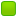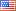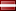###Author Topic: Output two digit numbers to the screen  (Read 16855 times)

####Slash5331

• Jr. Member
•• Posts: 9##### Output two digit numbers to the screen
« on: October 11, 2013, 04:50:09 AM »
Ok, I can convert character input to integers using

Code: [Select]
`mov ecx, dword[input]` where input is a .bss variable

And I have written the following program which is meant to convert an int to a character (so if the int is 14, it prints 14).  But it only works with 1 digit numbers, otherwise it just prints the ascii character.

Code: [Select]
`section .datasection .bssoutputbuffer resb 4section .textglobal _start_start:mov ecx, 8add ecx, 30hmov [outputbuffer], ecxmov ecx, outputbuffermov eax, 4mov ebx, 1mov edx, 4int 0x80mov eax, 1mov ebx, 0int 0x80`
also, when converting from char to integer, what is the purpose of the square brackets and dword?

####Frank Kotler

• NASM Developer
• Hero Member
•• Posts: 2658
• Country:##### Re: Output two digit numbers to the screen
« Reply #1 on: October 11, 2013, 05:34:54 AM »
Two digits only?
Code: [Select]
`mov al, 14aamadd ax, 3030hmov [outputbuffer], ahmov [outputbuffer + 1], almov ecx, outputbuffermov edx, 2mov ebx, 1 ; stdoutmov eax, 4 ; __NR_writeint 80hmov eax, 1 ; __NR_exitmov bl, 14 ; exitcode - can view with "echo \$?"int 80h`
The square brackets indicate "[contents of memory]". A variable name without the brackets indicates the address (offset part of the address) of the variable. "dword" is just the size - 4 bytes, 32 bits. If Nasm can tell the size from the size of the register involved, you don't need it...
Code: [Select]
`mov [outputbuffer + 1], al ; Nasm knows the sizemov byte [outputbuffer + 2], 10 ; Nasm needs to be told the size`
Best,
Frank

####Slash5331

• Jr. Member
•• Posts: 9##### Re: Output two digit numbers to the screen
« Reply #2 on: October 11, 2013, 06:33:06 AM »
Thanks very much, but as I'm very new to assembly there is a lot of this code I don't understand.  You explained the square brackets very well, but I am not familiar with 'aam', and why do you add 3030h to ax?
I vaguely understand:

mov [outputbuffer], ah
mov [outputbuffer + 1], al

but I'm not completely sure, i assume [outputbuffer] is the first digit, while [outputbuffer+1] is the second, but I don't understand the purpose of moving them into ah and al.

Also, how would one go about printing any number to the screen, regardless of digits?  Or is this a very complex process? if it is too complex I will simply wait and learn it later when I have a better understanding of nasm.

####Slash5331

• Jr. Member
•• Posts: 9##### Re: Output two digit numbers to the screen
« Reply #3 on: October 11, 2013, 07:04:43 AM »
OK nevermind, I actually understand all of that code now, but now I am wondering, if you have data in a 32 bit register ecx, how can one transfer that data to the low byte of the accumulator register?

obviously
mov, al, ecx
does not work.

####encryptor256

• Full Member
•• Posts: 250
• Country:• Win64 .##### Re: Output two digit numbers to the screen
« Reply #4 on: October 11, 2013, 07:27:57 AM »
Like this maybe:

mov al,cl
Encryptor256's Investigation \ Research Department.

####Frank Kotler

• NASM Developer
• Hero Member
•• Posts: 2658
• Country:##### Re: Output two digit numbers to the screen
« Reply #5 on: October 11, 2013, 06:45:39 PM »
Thanks A.K. Encryptor256! Yeah, we could get 32 bits of data into an 8-bit register... 8 bits at a time...
Code: [Select]
`mov al, cl; do something with itmov al, ch; do something with itshr ecx, 16mov al, cl; do something with itmov al, ch; do something with it`That probably isn't something you'd want to do. If the 32 bits of data in the 32-bit register is the address of something interesting, we could do:
Code: [Select]
`mov al, [ecx] ; get a byte of "[contents of memory]" from address in ecx into al`That's something you might want to do in the course of displaying a number... which gets us back on topic!

This "how do I print a number?" has got to be the champion "most frequently asked question" of all time, and I'm getting a bit "burnt out" by it. I apologize for showing you that "aam trick". It was a literal answer to the "two digits" part of your question, but not generally useful (good for "time numbers" maybe).

More generally, we need to multiply by ten or divide by ten. The "mul" and "div" instructions are a bit tricky in that some of the operands are "not shown", and the implicit operands and where the result comes vary with the size of the operand we do show. "div" is especially bad in that it'll crash your program if you screw it up. DOS used to report "divide by zero error" (I didn't divide by zero!) and Linux used to report "floating point error" (I didn't use floating point!). The actual exception is a "divide overflow exception": "div" doesn't do a 32-bit by 32-bit divide as you might expect, but a 64-bit (edx:eax) by 32-bit divide, and if the result doesn't fit in 32 bits it causes a processor exception. We may not care what's in edx, but the CPU does! (other sizes for other sizes of the "shown" operand)

The other "difficulty" is that if we divide 1234 by ten, we get a quotient of 123 and a remainder (in edx for a 32-bit "div") of 4, divide by ten again we get 12 and a remainder of 3, again - 1 remainder 2, and again - 0 remainder 1. At this point we're done (can stop when the quotient is less than ten and save a "div" if you want to). We get these remainders in the opposite order than we want to print them. There are various ways to deal with that - push 'em on the stack and pop 'em off, start at the "end" of the buffer and work toward the start, or put 'em in the buffer in "opposite" order and do a "string reverse" at the end... We will need to "convert" each of these numbers to a "character representing the number" by adding '0' or 48 or 30h to each before printing them, but you know how to do that part.

Going the other way, from "text representing a number" (the only kind of input we can get) to the "number" is just kinda "backwards". Start with a "result so far"of zero, get a character (leftmost first), "convert" it to a number by subtracting '0' or 48 or 30h, and - when we're sure it's a valid digit - multiply the "result so far" by ten and add in our new digit... until done.

There's some discussion of various ways to do this, and example code, here:
http://forum.nasm.us/index.php?topic=1514.msg6233#msg6233

We can get into this more if need be. It's an important - and very common - question.

When it comes to floating point numbers, just use "scanf" and "printf". They use the same instructions we would use, of course, but it gets "complex"...

Best,
Frank